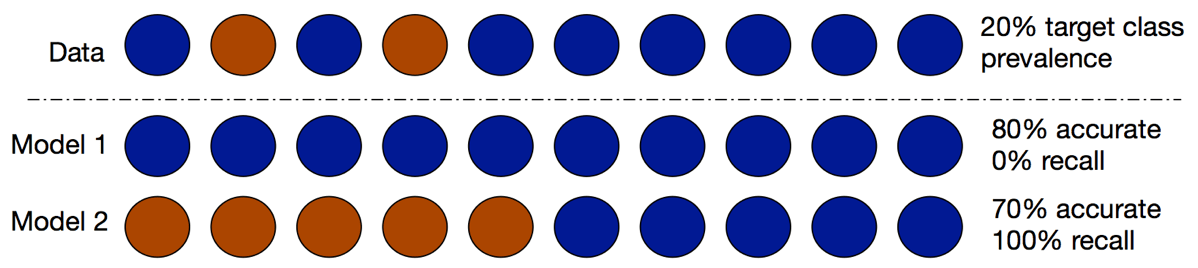# A bit on the F1 score floor

At Strata+Hadoop World “R Day” Tutorial, Tuesday, March 29 2016, San Jose, California we spent some time on classifier measures derived from the so-called “confusion matrix.”

We repeated our usual admonition to not use “accuracy itself” as a project quality goal (business people tend to ask for it as it is the word they are most familiar with, but it usually isn’t what they really want).One reason not to use accuracy: an example where a classifier that does nothing is “more accurate” than one that actually has some utility. (Figure credit Nina Zumel, slides here)

And we worked through the usual bestiary of other metrics (precision, recall, sensitivity, specificity, AUC, balanced accuracy, and many more).

We surveyed over a dozen common measures the data scientist is expected to know. But we found you could break them down into a manageable set if you organized them as follows:

• Is the measure attempting to value a situation (such as characterizing positive predictions) or the quality of the classifier (such as AUC)?
• Which errors is the measure more sensitive to: FalsePositives, FalseNegatives, or a mixture?
• Is the measure sensitive to population prevalence or not?

While this may seem complicated, this is much better than the traditions used when trying to estimate inter-observer or tagger agreement (where there are around 100 measures, many of which combine effect size and significance, and requires significant research to understand which measures are monotone related to each other; see: Warrens, M. (2008). “On similarity coefficients for 2× 2 tables and correction for chance.” Psychometrika, 73(3), 487–502).

Another issue is the crypto-synonymity of the number of measures. One can be expected to remember: Sensitivity==Recall (which one you use giving away where you come from!). But one also should remember:

• True Positive Rate == Recall
• 1 – (False Negative Rate) == True Positive Rate
• Postive Predictive Value == Precision

Though I’d hate to argue the last one without my notes as:

• Positive Predictive Value is defined as: (sensitivity * Prevalence)/((sensitivity*Prevalence) + ((1-specificity)*(1-Prevalence)))
• Precision is defined as: TruePositives/(FalsePositives + TruePositives)

And then we ran into some exotic measures and relations between. For a classifier that only returns two scores we can define AUC as the area under the ROC plot given by the following diagram:In this case we have:

• AUC == Balanced Accuracy
• AUC == Probability a positive example scores above a negative example (with ties counted as 1/2, let’s call this the “probability game”).

The last few were complicated enough that we ended up using Python’s symbolic math package symPy to check the algebra (though we called it from R using rSymPy). We share our work here.

But we had left out F1. Is F1 monotone related to any of the other measures? Maybe the complicated AUC/BalancedAccuracy/ProbabilityGame cluster? Of course F1 is sensitive to prevalence (population distribution) and AUC is not, so F1 and AUC can’t be that closely related. But it turns out they are pretty different (that is not to say either is evidence the other is wrong, as any 1-dimensional summary of the confusion matrix is going to leave a lot out).

We ended up finding a corner case that really shows the difference between AUC and F1.

Consider a classifier that never says “no.” By convention this classifier has an AUC of 1/2 which properly signals its uselessness. But through some algebra we can show the F1 of such a classifier is `2*Prevalence/(1 + Prevalence)`. This makes sense- as prevalence goes to 1 the accuracy of the “never say no” classifier also goes to 1, and we would expect a population sensitive measure like F1 to reward that. This is also why it is important to know your population prevalence even if you are using modeling methods that actually tolerate imbalance. What one is perceiving as modeling failure may just be scoring failure (such as scoring at different thresholds and accidentally doing nothing more than moving around the ROC curve).

Let’s end with an example. When scoring the “never say no” classifier on a population that is 75% positive you get an F1 score of 0.857 just for showing up (and this F1 is bigger than something simple like 0.75)! I am sure everyone knows to “balance classes”, but I doubt many of us remember the F1 “score floor” as being so high in this case. Also note: F1 is scale invariant- so increasing test set size does not work around this issue.

Tagged as:### jmount

Data Scientist and trainer at Win Vector LLC. One of the authors of Practical Data Science with R.